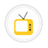Least common multipleTo find Least common multiple of two numbers.Heat TransferThere are three methods of thermal energy transfer: conduction, convection, and radiation.Conduction of electricity through acids and basesConduction of electricity through acids and basesFinancial LiiteracyFinancial LiiteracyAlgebraic Identity (a+b)²The identity (a+b)2 = a2 + 2ab + b2 is verified by cutting and pasting of paper. This identity can be verified geometrically by taking other values of a and b.Factorization Of PolynomialTo factorise a polynomial, say (x2 + 4x + 3)Solving a linear equationTo solve a linear equation, say 2x + 3 = 5To find fractions equivalent to a given fraction​To find fractions equivalent to a given fraction​Sum of fractions with same denominatorsTo find the sum of fractions with same denominators [say 1/5 + 3/5]​Subtraction of fractions with same denominatorTo subtract a smaller fraction from greater fraction with the same denominator.Geography LabGeography LabTangent - Radius PropertyTo verify experimentally that the tangent at any point to a circle is perpendicular to the radius through that point.Clause IdentificationClause IdentificationITS MathsITS MathsSet theoretic operations using Venn diagramsTo represent set theoretic operations using Venn diagramsSet Theory: A ∪ (B ∩ C) = (A ∪ B) ∩ (A ∪ C)To verify distributive law for three given non-empty sets A, B and C, that is, A ∪ (B ∩ C) = (A ∪ B) ∩ (A ∪ C)Cyclic Quadrilateral PropertyTo verify that the opposite angles of a cyclic quadrilateral are supplementary.Area of a triangles - same base and same parallelsTo verify that the triangles on the same base and between the same parallels are equal in area.Exterior angle property of a triangleTheoram to proof the exterior angle property of triangleObservation of Micro-OrganismsObservation of micro-organism in wateEvaporationExperiment for evaporationArchimedes PrincipleExperiment for Archimedes principlesSingle displacement reactionExperiment for Single displacement reactionThe world: continents and oceansThe world: continents and oceansSpring tides and neap tideSpring tidesa and neap tideLayers of the atmosphereLayers of the atmosphereWater cycleWater cycleIndia's Natural VegetationIndia's Natural VegetationAlgebraic Identity (a-b)³To verify the algebraic identity (a-b)³ = a³-b³-3ab(a-b)Algebraic Identity (a + b)³To verify the algebraic identity: (a + b)³ = a³ + b³ + 3a²b + 3ab²Angle in a semicircleTo verify that angle in a semi-circle is a right angle, using vector methodAngle sum property of triangleTo verify sum of all interior angles of a triangle is 180°Surface area and volume of coneTo form a cone from a sector of a circle and to find the formula for its curved surface areaAlgebraic Identity (a²-b²)To verify algebraic identity a²-b²=(a+b)(a-b)Ratio of circumference and diameter of circleTo find the ratio of circumference and diameter of a circleDistributive vector multiplicationTo verify the distributive property of vector multiplicationAlgebraic Identity (a³+b³)To verify the algebraic identity a³+b³=(a+b)(a²-ab+b²)Sum of fractions with different denominatorsTo find sum of unlike fractionAddition of algebraic expressionTo add two algebraic expressionsAlgebraic Identity (a³ - b³)To verify the algebraic identity a³- b³Algebraic Identity (a - b)²To verify the algebraic identity (a - b)²Algebraic Identity (a+b+c)²To verify the algebraic identity (a + b + c)²Congruence of trianglesTo verify experimentally the different rules for congruence of trianglesCube and its surface areaTo form a cube and find the formula for its surface areaCuboid and its total surface areaTo form a cuboid and find the formula for its total surface areaFactorization of Polynomial 2x² +4xTo factorise a polynomial 2x² + 4xFrustom of a coneTo form a frustum of a coneMultiply two linear algebraic expressionsTo multiply two linear algebraic expressionsPythagoras theorem by bhaskara methodTo verify the pythagoras theorem by Bhaskara methodRelation and FunctionTo identify Relation and FunctionPerimeter of rectangle and area of squareTo verify that amongst all the rectangles of the same perimeter, the square has the maximum areaSimilarity of two trianglesTo establish the criteria for similarity of two trianglesSubtraction of fractions with different denominatorTo subtract a smaller fraction ​from greater fraction with different denominatorsSurface area and the volume of a frustum of a coneTo obtain formulae for the surface area and the volume of a frustum of a coneVolume of a cuboidTo obtain a formula for the volume of a cuboidTo subtract a polynomial from another polynomialTo subtract a polynomial from another polynomialTo make a square by paper folding and cuttingTo make a square by paper folding and cutting